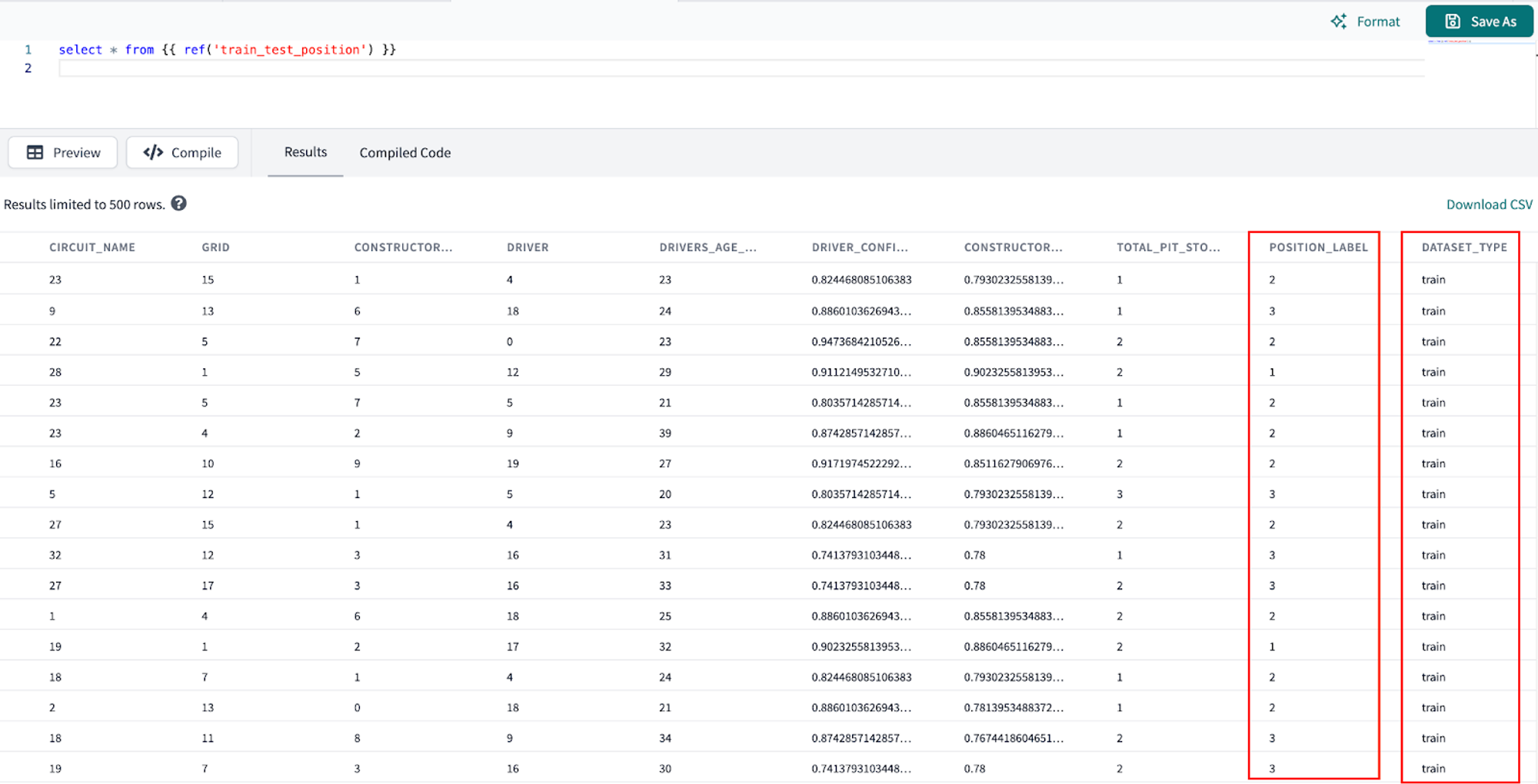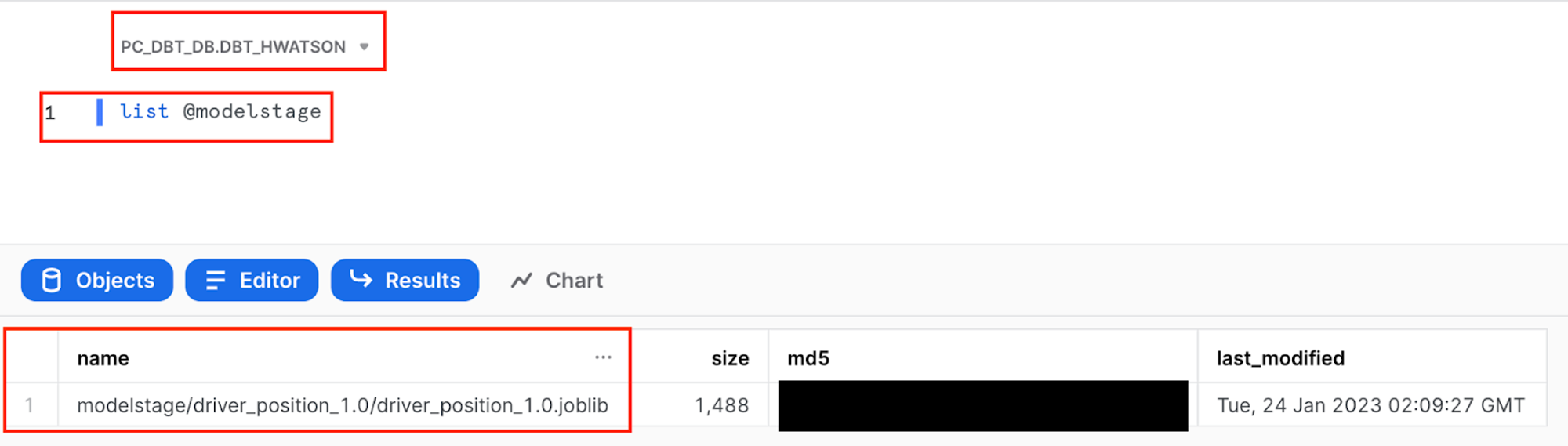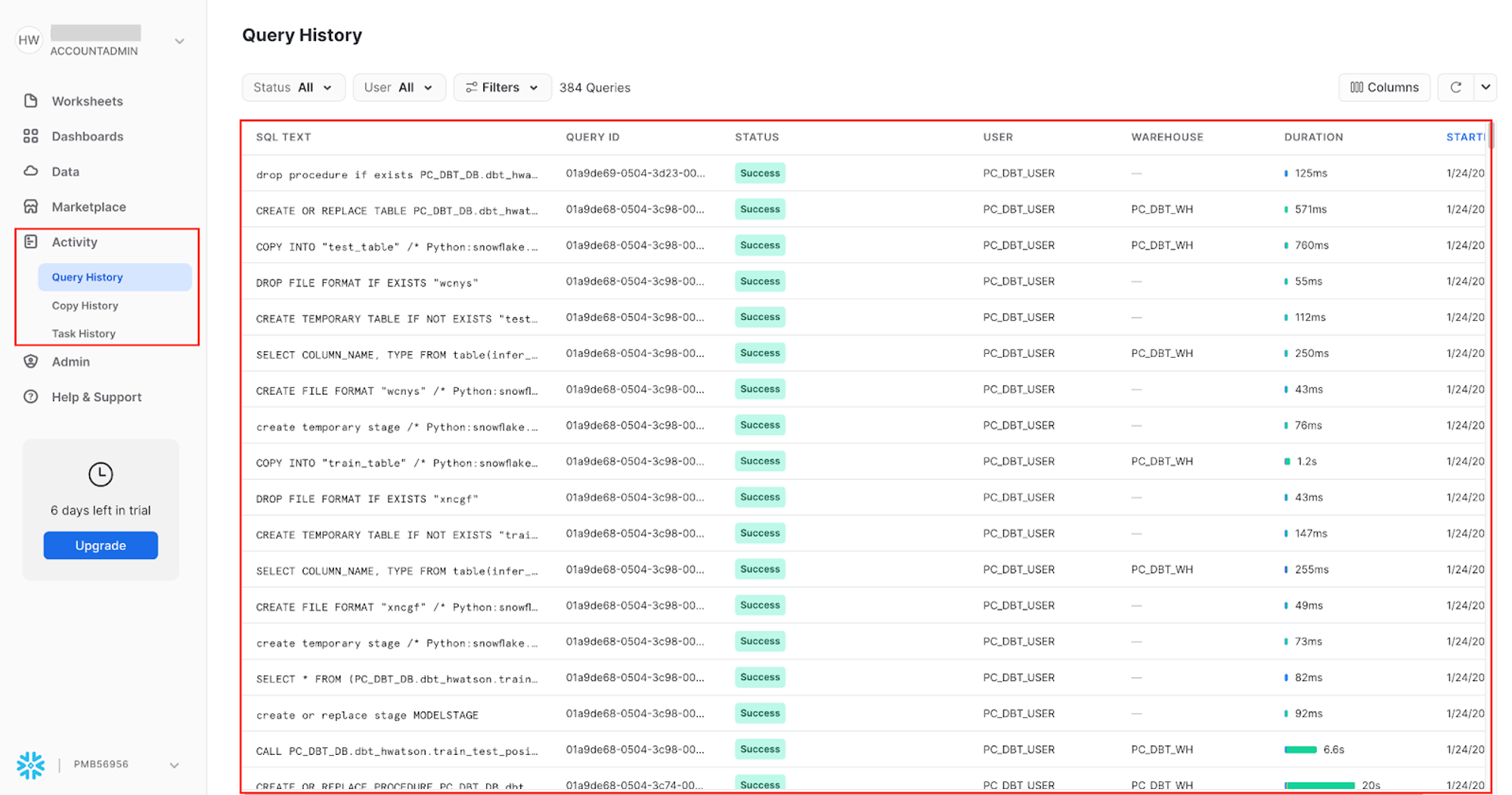# Machine Learning: training and prediction

We’re ready to start training a model to predict the driver’s position. Now is a good time to pause and take a step back and say, usually in ML projects you’ll try multiple algorithms during development and use an evaluation method such as cross validation to determine which algorithm to use. You can definitely do this in your dbt project, but for the content of this lab we’ll have decided on using a logistic regression to predict position (we actually tried some other algorithms using cross validation outside of this lab such as k-nearest neighbors and a support vector classifier but that didn’t perform as well as the logistic regression and a decision tree that overfit).

There are 3 areas to break down as we go since we are working at the intersection all within one model file:

1. Machine Learning
2. Snowflake and Snowpark
3. dbt Python models

If you haven’t seen code like this before or use joblib files to save machine learning models, we’ll be going over them at a high level and you can explore the links for more technical in-depth along the way! Because Snowflake and dbt have abstracted away a lot of the nitty gritty about serialization and storing our model object to be called again, we won’t go into too much detail here. There’s a lot going on here so take it at your pace!

## Training and saving a machine learning model​

1. Project organization remains key, so let’s make a new subfolder called train_predict under the ml folder.

2. Now create a new file called train_test_position and copy and save the following code:

import snowflake.snowpark.functions as Ffrom sklearn.model_selection import train_test_splitimport pandas as pdfrom sklearn.metrics import confusion_matrix, balanced_accuracy_scoreimport iofrom sklearn.linear_model import LogisticRegressionfrom joblib import dump, loadimport joblibimport loggingimport sysfrom joblib import dump, loadlogger = logging.getLogger("mylog")def save_file(session, model, path, dest_filename):    input_stream = io.BytesIO()    joblib.dump(model, input_stream)    session._conn.upload_stream(input_stream, path, dest_filename)    return "successfully created file: " + pathdef model(dbt, session):    dbt.config(        packages = ['numpy','scikit-learn','pandas','numpy','joblib','cachetools'],        materialized = "table",        tags = "train"    )    # Create a stage in Snowflake to save our model file    session.sql('create or replace stage MODELSTAGE').collect()    #session._use_scoped_temp_objects = False    version = "1.0"    logger.info('Model training version: ' + version)    # read in our training and testing upstream dataset    test_train_df = dbt.ref("train_test_dataset")    #  cast snowpark df to pandas df    test_train_pd_df = test_train_df.to_pandas()    target_col = "POSITION_LABEL"    # split out covariate predictors, x, from our target column position_label, y.    split_X = test_train_pd_df.drop([target_col], axis=1)    split_y = test_train_pd_df[target_col]    # Split out our training and test data into proportions    X_train, X_test, y_train, y_test  = train_test_split(split_X, split_y, train_size=0.7, random_state=42)    train = [X_train, y_train]    test = [X_test, y_test]    # now we are only training our one model to deploy    # we are keeping the focus on the workflows and not algorithms for this lab!    model = LogisticRegression()    # fit the preprocessing pipeline and the model together     model.fit(X_train, y_train)       y_pred = model.predict_proba(X_test)[:,1]    predictions = [round(value) for value in y_pred]    balanced_accuracy =  balanced_accuracy_score(y_test, predictions)    # Save the model to a stage    save_file(session, model, "@MODELSTAGE/driver_position_"+version, "driver_position_"+version+".joblib" )    logger.info('Model artifact:' + "@MODELSTAGE/driver_position_"+version+".joblib")    # Take our pandas training and testing dataframes and put them back into snowpark dataframes    snowpark_train_df = session.write_pandas(pd.concat(train, axis=1, join='inner'), "train_table", auto_create_table=True, create_temp_table=True)    snowpark_test_df = session.write_pandas(pd.concat(test, axis=1, join='inner'), "test_table", auto_create_table=True, create_temp_table=True)    # Union our training and testing data together and add a column indicating train vs test rows    return  snowpark_train_df.with_column("DATASET_TYPE", F.lit("train")).union(snowpark_test_df.with_column("DATASET_TYPE", F.lit("test")))
3. Execute the following in the command bar:

dbt run --select train_test_position
4. Breaking down our Python script here:

• We’re importing some helpful libraries.
• Defining a function called save_file() that takes four parameters: session, model, path and dest_filename that will save our logistic regression model file.
• session an object representing a connection to Snowflake.
• model an object that needs to be saved. In this case, it's a Python object that is a scikit-learn that can be serialized with joblib.
• path a string representing the directory or bucket location where the file should be saved.
• dest_filename a string representing the desired name of the file.
• Creating our dbt model
• Within this model we are creating a stage called MODELSTAGE to place our logistic regression joblib model file. This is really important since we need a place to keep our model to reuse and want to ensure it's there. When using Snowpark commands, it's common to see the .collect() method to ensure the action is performed. Think of the session as our “start” and collect as our “end” when working with Snowpark (you can use other ending methods other than collect).
• Using .ref() to connect into our train_test_dataset model.
• Now we see the machine learning part of our analysis:
• Create new dataframes for our prediction features from our target variable position_label.
• Split our dataset into 70% training (and 30% testing), train_size=0.7 with a random_state specified to have repeatable results.
• Specify our model is a logistic regression.
• Fit our model. In a logistic regression this means finding the coefficients that will give the least classification error.
• Round our predictions to the nearest integer since logistic regression creates a probability between for each class and calculate a balanced accuracy to account for imbalances in the target variable.
• Right now our model is only in memory, so we need to use our nifty function save_file to save our model file to our Snowflake stage. We save our model as a joblib file so Snowpark can easily call this model object back to create predictions. We really don’t need to know much else as a data practitioner unless we want to. It’s worth noting that joblib files aren’t able to be queried directly by SQL. To do this, we would need to transform the joblib file to an SQL querable format such as JSON or CSV (out of scope for this workshop).
• Finally we want to return our dataframe, but create a new column indicating what rows were used for training and those for training.
5. Viewing our output of this model:Preview which rows of our model were used for training and testing
6. Let’s pop back over to Snowflake and check that our logistic regression model has been stored in our MODELSTAGE using the command:

list @modelstageList the objects in our Snowflake stage to check for our logistic regression to predict driver position
7. To investigate the commands run as part of train_test_position script, navigate to Snowflake query history to view it Activity > Query History. We can view the portions of query that we wrote such as create or replace stage MODELSTAGE, but we also see additional queries that Snowflake uses to interpret python code.View Snowflake query history to see how python models are run under the hood

## Predicting on new data​

1. Create a new file called predict_position and copy and save the following code:

import loggingimport joblibimport pandas as pdimport osfrom snowflake.snowpark import types as TDB_STAGE = 'MODELSTAGE'version = '1.0'# The name of the model filemodel_file_path = 'driver_position_'+versionmodel_file_packaged = 'driver_position_'+version+'.joblib'# This is a local directory, used for storing the various artifacts locallyLOCAL_TEMP_DIR = f'/tmp/driver_position'DOWNLOAD_DIR = os.path.join(LOCAL_TEMP_DIR, 'download')TARGET_MODEL_DIR_PATH = os.path.join(LOCAL_TEMP_DIR, 'ml_model')TARGET_LIB_PATH = os.path.join(LOCAL_TEMP_DIR, 'lib')# The feature columns that were used during model training# and that will be used during predictionFEATURE_COLS = [        "RACE_YEAR"        ,"CIRCUIT_NAME"        ,"GRID"        ,"CONSTRUCTOR_NAME"        ,"DRIVER"        ,"DRIVERS_AGE_YEARS"        ,"DRIVER_CONFIDENCE"        ,"CONSTRUCTOR_RELAIBLITY"        ,"TOTAL_PIT_STOPS_PER_RACE"]def register_udf_for_prediction(p_predictor ,p_session ,p_dbt):    # The prediction udf    def predict_position(p_df: T.PandasDataFrame[int, int, int, int,                                        int, int, int, int, int]) -> T.PandasSeries[int]:        # Snowpark currently does not set the column name in the input dataframe        # The default col names are like 0,1,2,... Hence we need to reset the column        # names to the features that we initially used for training.        p_df.columns = [*FEATURE_COLS]            # Perform prediction. this returns an array object        pred_array = p_predictor.predict(p_df)        # Convert to series        df_predicted = pd.Series(pred_array)        return df_predicted    # The list of packages that will be used by UDF    udf_packages = p_dbt.config.get('packages')    predict_position_udf = p_session.udf.register(        predict_position        ,name=f'predict_position'        ,packages = udf_packages    )    return predict_position_udfdef download_models_and_libs_from_stage(p_session):    p_session.file.get(f'@{DB_STAGE}/{model_file_path}/{model_file_packaged}', DOWNLOAD_DIR)def load_model(p_session):    # Load the model and initialize the predictor    model_fl_path = os.path.join(DOWNLOAD_DIR, model_file_packaged)    predictor = joblib.load(model_fl_path)    return predictor# -------------------------------def model(dbt, session):    dbt.config(        packages = ['snowflake-snowpark-python' ,'scipy','scikit-learn' ,'pandas' ,'numpy'],        materialized = "table",        tags = "predict"    )    session._use_scoped_temp_objects = False    download_models_and_libs_from_stage(session)    predictor = load_model(session)    predict_position_udf = register_udf_for_prediction(predictor, session ,dbt)    # Retrieve the data, and perform the prediction    hold_out_df = (dbt.ref("hold_out_dataset_for_prediction")        .select(*FEATURE_COLS)    )    # Perform prediction.    new_predictions_df = hold_out_df.withColumn("position_predicted"        ,predict_position_udf(*FEATURE_COLS)    )    return new_predictions_df
2. Execute the following in the command bar:

dbt run --select predict_position
3. Commit and push our changes to keep saving our work as we go using the commit message logistic regression model training and application before moving on.

4. At a high level in this script, we are:

• Retrieving our staged logistic regression model
• Placing the model within a user defined function (UDF) to call in line predictions on our driver’s position
5. At a more detailed level:

• Import our libraries.
• Create variables to reference back to the MODELSTAGE we just created and stored our model to.
• The temporary file paths we created might look intimidating, but all we’re doing here is programmatically using an initial file path and adding to it to create the following directories:
• LOCAL_TEMP_DIR ➡️ /tmp/driver_position
• TARGET_MODEL_DIR_PATH ➡️ /tmp/driver_position/ml_model
• TARGET_LIB_PATH ➡️ /tmp/driver_position/lib
• Provide a list of our feature columns that we used for model training and will now be used on new data for prediction.
• Next, we are creating our main function register_udf_for_prediction(p_predictor ,p_session ,p_dbt):. This function is used to register a user-defined function (UDF) that performs the machine learning prediction. It takes three parameters: p_predictor is an instance of the machine learning model, p_session is an instance of the Snowflake session, and p_dbt is an instance of the dbt library. The function creates a UDF named predict_churn which takes a pandas dataframe with the input features and returns a pandas series with the predictions.
• ⚠️ Pay close attention to the whitespace here. We are using a function within a function for this script.
• We have 2 simple functions that are programmatically retrieving our file paths to first get our stored model out of our MODELSTAGE and downloaded into the session download_models_and_libs_from_stage and then to load the contents of our model in (parameters) in load_model to use for prediction.
• Take the model we loaded in and call it predictor and wrap it in a UDF.
• Return our dataframe with both the features used to predict and the new label.

🧠 Another way to read this script is from the bottom up. This can help us progressively see what is going into our final dbt model and work backwards to see how the other functions are being referenced.

1. Let’s take a look at our predicted position alongside our feature variables. Open a new scratchpad and use the following query. I chose to order by the prediction of who would obtain a podium position:
select * from {{ ref('predict_position') }} order by position_predicted
2. We can see that we created predictions in our final dataset, we are ready to move on to testing!
0